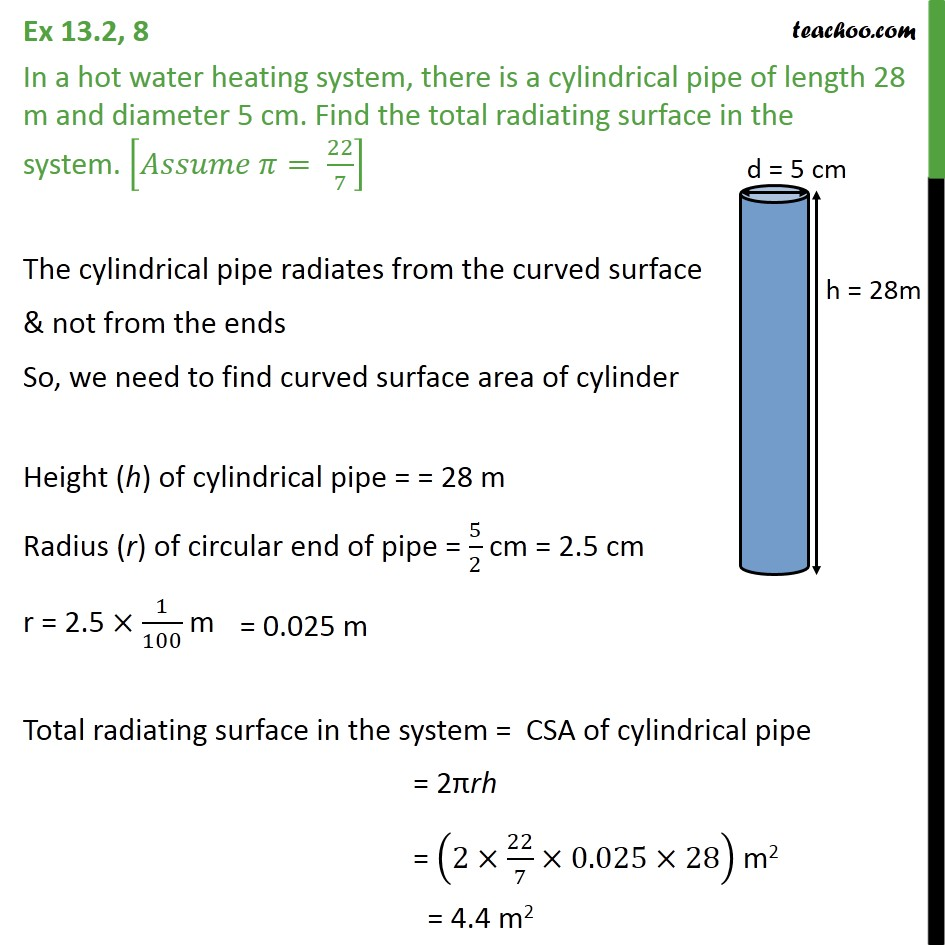Area Of Cylinder

Chapter 11 Class 9 Surface Areas and Volumes
Concept wiseLearn in your speed, with individual attention - Teachoo Maths 1-on-1 Class

### Transcript

Question 8 In a hot water heating system, there is a cylindrical pipe of length 28 m and diameter 5 cm. Find the total radiating surface in the system. [ = 22/7] The cylindrical pipe radiates from the curved surface & not from the ends So, we need to find curved surface area of cylinder Height (h) of cylindrical pipe = 28 m Radius (r) of circular end of pipe = 5/2 cm = 2.5 cm r = 2.5 1/100 m Total radiating surface in the system = CSA of cylindrical pipe = 2 rh = (2 22/7 0.025 28) m2 = 4.4 m2